Question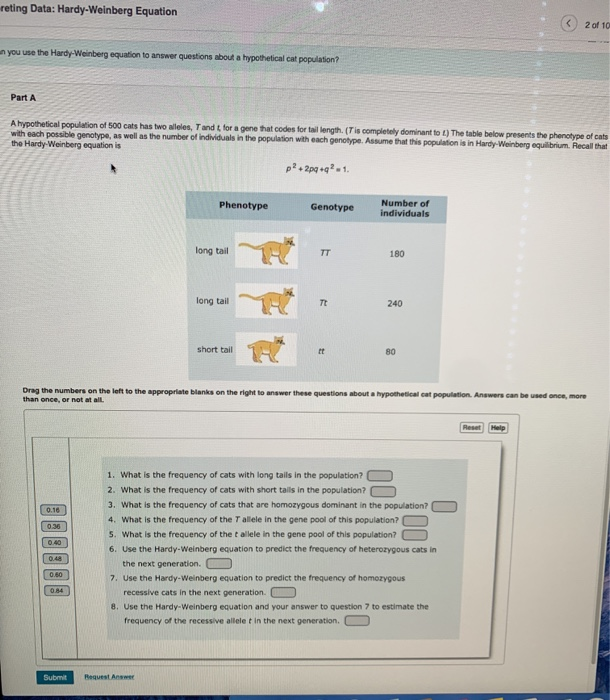Please look in the image for solution: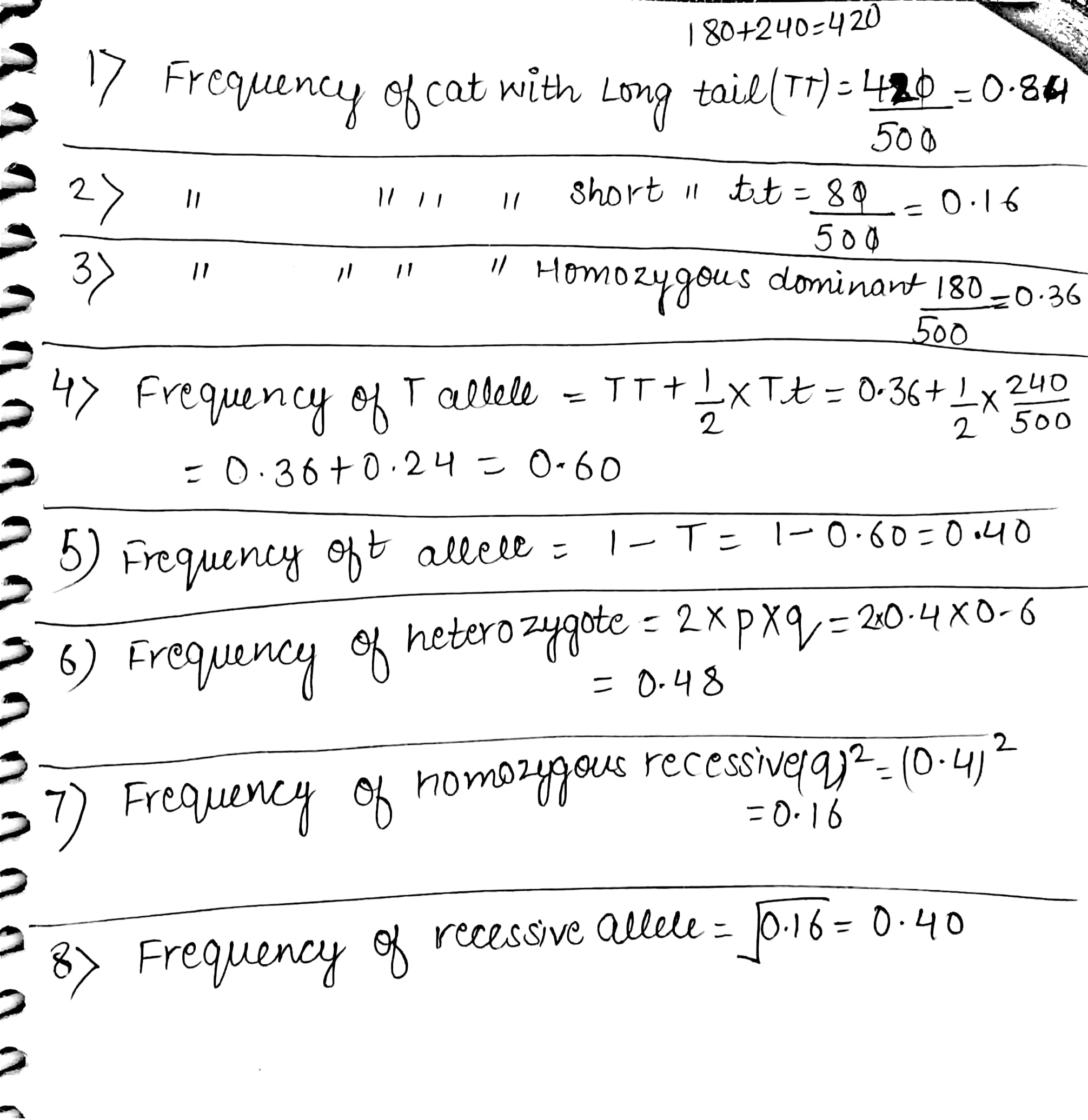#### Earn Coins

Coins can be redeemed for fabulous gifts.

Similar Homework Help Questions
• ### Evolution 1. Assume this population is in Hardy-Weinberg equilibrium. In a population of 120 cats, 35...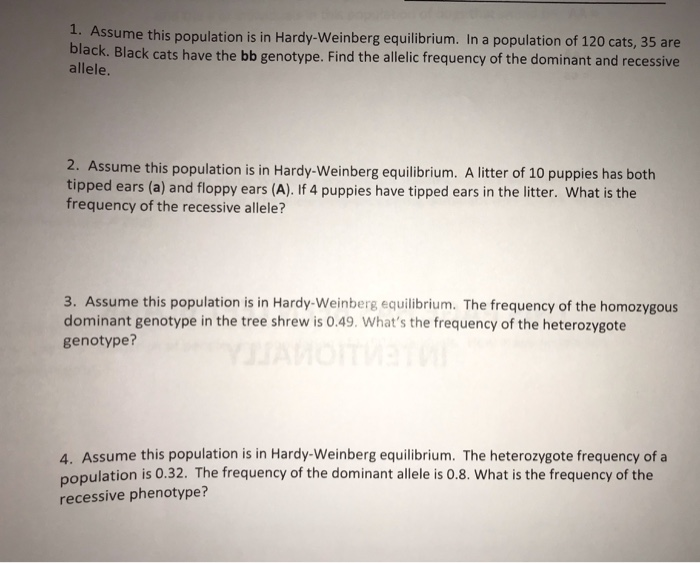Evolution 1. Assume this population is in Hardy-Weinberg equilibrium. In a population of 120 cats, 35 are black. Black cats have the bb genotype. Find the allelic frequency of the dominant and recessive allele. 2. Assume this population is in Hardy-Weinberg equilibrium. A litter of 10 puppies has both tipped ears (a) and floppy ears (A). If 4 puppies have tipped ears in the litter. What is the frequency of the recessive allele? 3. Assume this population is in Hardy-Weinberg...

• ### (Hardy Weinberg Equation) In a population of rabbits, there are 423 A1A1, 1484 A2A2, and 1661...

(Hardy Weinberg Equation) In a population of rabbits, there are 423 A1A1, 1484 A2A2, and 1661 A1A2 individuals. If the enviornment changes so that the homozygous recessive genotype suffers a reduction of fitness where it's fitness is now 0.59 , but the other genotypes are unaffected, what will be the frequency of the dominant allele in the NEXT generation?

• ### The Hardy-Weinberg principle and its equations predict that frequencies of alleles and genotypes remain constant from generation to generation in populations that are not evolving

.1. The Hardy-Weinberg principle and its equations predict that frequencies of alleles and genotypes remain constant from generation to generation in populations that are not evolving. What five conditions does this prediction assume to be true about such a population? a._______  b._______  c._______  d._______  e._______  2. Before beginning the activity, answer the following general Hardy-Weinberg problems for practice (assume that the population is at Hardy-Weinberg equilibrium).a. If the frequency of a recessive allele is 0.3, what is the frequency of the dominant...

• ### 2.3 Problem 3 The Hardy-Weinberg equation is useful for predicting the percent of a hu- man...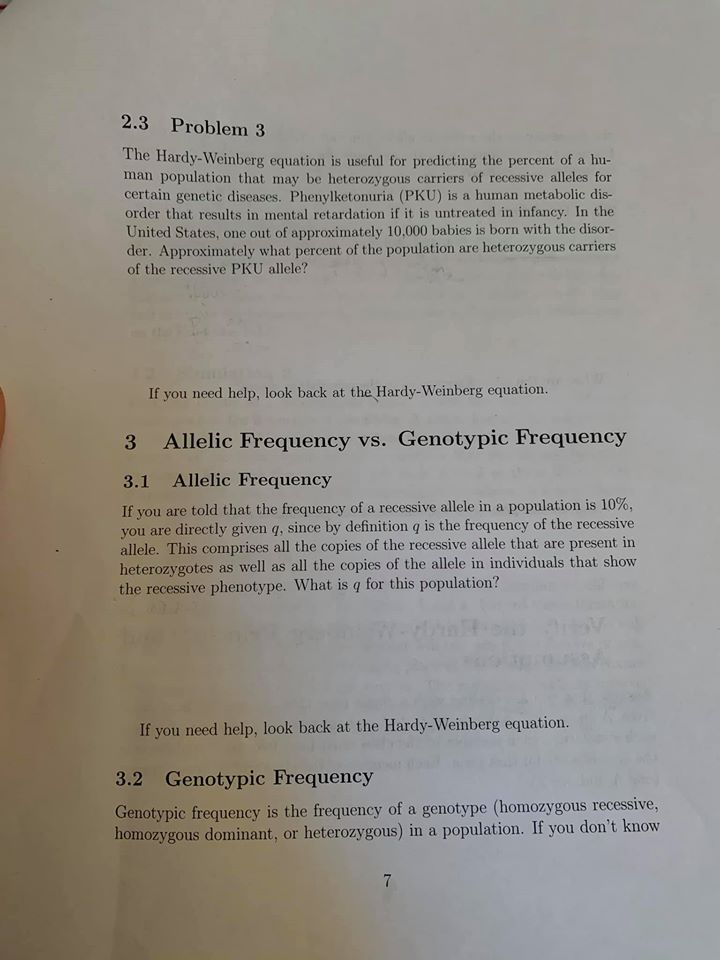2.3 Problem 3 The Hardy-Weinberg equation is useful for predicting the percent of a hu- man population that may be heterozygous carriers of recessive alleles for certain genetic diseases. Phenylketonuria (PKU) is a human metabolic dis- order that results in mental retardation if it is untreated in infancy. In the United States, one out of approximately 10.000 babies is born with the disor- der. Approximately what percent of the population are heterozygous carriers of the recessive PKU allele? If you...

• ### Testing the goodness of fit between the data and the Hardy Weinberg equilibrium model generated expectations....

Testing the goodness of fit between the data and the Hardy Weinberg equilibrium model generated expectations. 4.1 In a species of bird, feather color is controlled by genes at a single locus, with the red feather allele dominant to the yellow feather allele. A population has 22 red and 14 yellow birds, with 9 of the red birds having a homozygous dominant genotype. Is this population in equilibrium? Calculate p and q from the number of individuals of each genotype:...

• ### EXERCISE 6 HARDY-WEINBERG EQUILIBRIUM Work in a small group or alone to complete this exercise. In...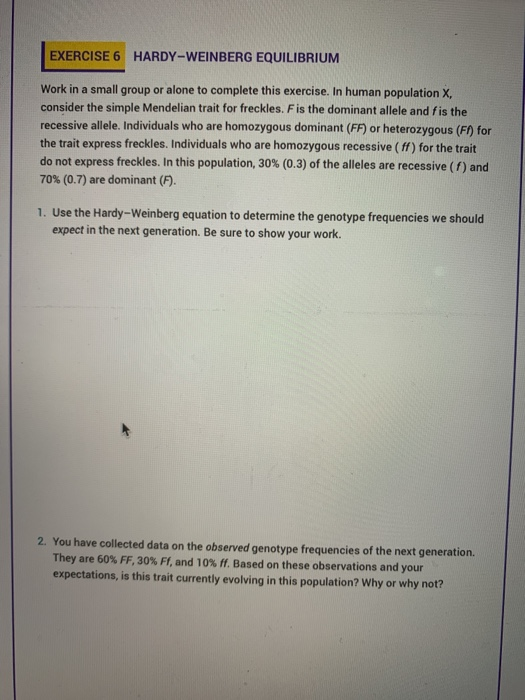EXERCISE 6 HARDY-WEINBERG EQUILIBRIUM Work in a small group or alone to complete this exercise. In human population X consider the simple Mendelian trait for freckles. F is the dominant allele and fis the recessive allele. Individuals who are homozygous dominant (FF) or heterozygous (F) for the trait express freckles. Individuals who are homozygous recessive (ff) for the trait do not express freckles. In this population, 30% (0.3) of the alleles are recessive (1) and 70% (0.7) are dominant (F)....

• ### 2. Hardy-Weinberg Equilibrium; chi-square test Sickle cell anemia is a recessive disorder caused by a recessive...

2. Hardy-Weinberg Equilibrium; chi-square test Sickle cell anemia is a recessive disorder caused by a recessive mutation (S) in the b-hemoglobin gene. 80% of affected SS individuals die before reproducing.   Heterozygotes (AS) and homozygous dominant (AA) individuals do not have sickle cell anemia. The table below shows the number of people of each genotype in a population of 100 people in population of Cameroon. Observed # individuals in a Cameroon population AA AS SS 62 32 6 What are the...

• ### 2. Assume a population is in Hardy-Weinberg equilibrium for a given genetic autosomal trait. What proportion...

2. Assume a population is in Hardy-Weinberg equilibrium for a given genetic autosomal trait. What proportion of individuals in the population are heterozygous for the gene if the frequency of the recessive allele is 1%.

• ### Which of the following statements regarding the Hardy-Weinberg Equation is TRUE? Answers: It can only be u...

Which of the following statements regarding the Hardy-Weinberg Equation is TRUE? Answers: It can only be used when allele and genotype frequencies remain constant across generations in a population It can only be used when allele and genotype frequencies change across generations in a population It has no assumptions It mathematically shows how recessive alleles in a population decrease over time It reveals when allele frequencies change over time in a population Assuming Hardy-Weinberg Equilibrium, what percentage of individuals in...

• ### (Hardy weinberg equation) You are examining a population that is under selection where the fitness of...

(Hardy weinberg equation) You are examining a population that is under selection where the fitness of the heterozygotes is 0.34, homozygotes is 1. If there's 546 AA, 127 aa, and 982 Aa individuals in population, what will be the "p" value for the next generation?